# An Introduction to Prim's Algorithm in Python

## Use Prim's Algorithm to find the Minimum Spanning Tree of an undirected graph.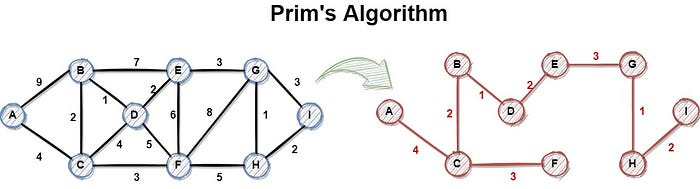Hi everyone, a couple of months ago, we discussed Kruskal's algorithm to find the Minimum Spanning Tree (MST) of a given graph. Today, we are going to examine another very well-known algorithm to find the MST, called Prim's algorithm. More specifically we are going to talk about the following topics:

``````1. Motivation
2. Pseudocode
3. Pen and Paper example
4. Python implementation
5. Example in Python
6. Conclusion
``````

We have a lot of stuff to cover, so let's get started.

### Motivation

Let me start with a hypothetical scenario. Let's imagine that we are the mayor of a small city and we want to improve the road network of the city. Our resources as regards human resources and money are limited. So we decide to find the most important points of interest and to improve the roads that connect them. To succeed, we must find the minimum number of roads' total length that must be improved, in order to be able to move from one point to any other point in the city, not necessarily by the shortest path. In other words, we choose to reconstruct a subset of roads, with the minimum total length that connects all the points. We can visualize the above city in a form of a graph, where each point of interest is represented as a Node (Vertex) and each road as an edge. Our graph has the following form.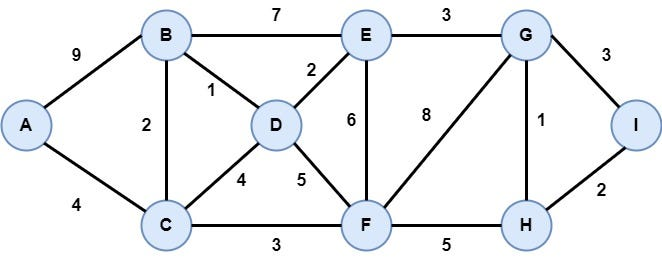What we need to do is to find the Minimum Spanning Tree (MST). In a brief, a tree is a graph without circles. An MST is a tree whose edges have the minimum total cost. As we already know, Kruskal's algorithm is one approach to creating the MST from a given graph. Today we are going to see Prim's algorithm to find the MST. Like Kruskal's algorithm, Prim's algorithm is a greedy algorithm and is used to find the MST in a weighted and undirected graph.

Graph Data Structure — Theory and Python Implementation

Prim's Algorithm takes a graph as an input and returns the Minimum Spanning Tree of that graph. To do that, it starts from a vertex arbitrarily, inserting it in an empty tree. After that, creates the tree step by step adding the vertex with the lowest distance from its neighbors that already belongs to the tree. Each vertex that is inserted on the tree, is removed from the graph. The whole procedure ends when there are no vertices in the graph. The pseudocode of Prim's algorithm is the following:

``````1   function Prim(_Graph_):
2      create a new empty tree F
3      create a list with all the vertices V
4      initialize the distance of each of node to infinity
5      (dist = inf)
6      Select a vertex X at random, remove it from V
7      and add it on tree F
8      for each neighbor n of X:
9         compute the distance from X to n
10        update the previous_vertex of n to X
11     while vertices are not empty:
12        v is the vertex with the lowest dist value
13        remove v from vertices
14        insert v into the tree
15        for each neighbor n of v that belongs to vertices:
16           compute the distance from v to n
17           if the dist value of v < the previous dist value:
18              update the dist value of v to the new dist value
19              update the previous vertex of n to v
20   return tree
``````

### Pen and Paper Example

Having an intuition about the algorithm, let's try to solve the problem we discussed above, regarding the reconstruction of the road network of a city. There are nine points of interest in the city that are represented as vertices in the graph and the roads between these points are represented as edges as follows:Step 1: Initialization

In this step, all the nodes (vertices) are inserted in the data structure (i.e. a list) vertices. Each element has the form (vertex, length from previous vertex, previous vertex). In this example, we assume that we start the construction of the Minimum Spanning Tree from Vertex A.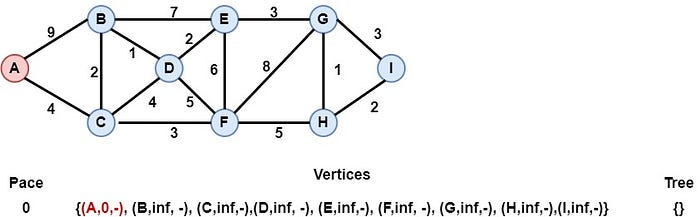Step 2: Vertex A is inserted into the tree

Vertex A has the lowest distance from its previous node (None), so it is inserted into the tree. After that, for each neighbor of A, that exists in the vertices, we calculate the distance from vertex A.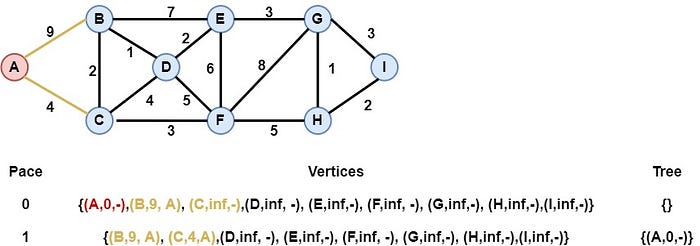Step 3: Vertex C is inserted into the tree

Vertex C has the lowest distance, so it is inserted into the tree. Similarly, for each neighbor of C, that exists in the vertices, we calculate and update the distance from vertex C. Note that through vertex C we can reach vertex B at a lower cost, so we update the details of vertex B.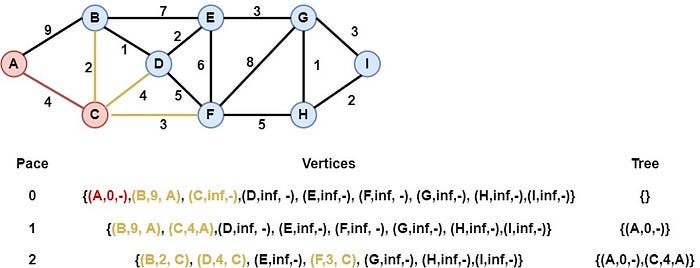Step 4: Vertex B is inserted into the tree

Vertex B has the lowest distance, so it is inserted into the tree and we calculate the distance for each of its neighbors that exist in vertices. As we can see we find a better way to reach vertex D, so we update the elements of vertex D.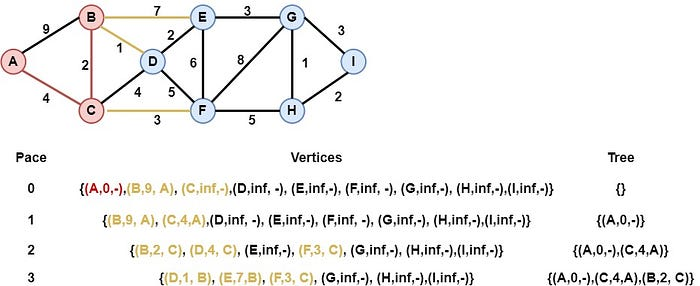Step 5: Vertex D is inserted into the tree

Vertex D is inserted into the tree as it has the lowest distance, while we find a better way to reach the vertex E through the vertex D.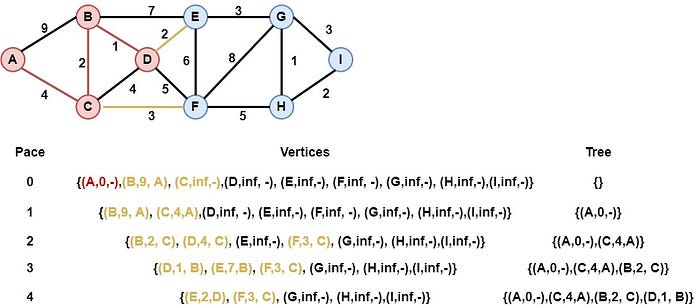Step 6: Vertex E is inserted into the tree

Vertex E is inserted into the tree, as it has the lowest distance. At the same time, we have found a way to reach the vertex G through vertex E with a cost of 3.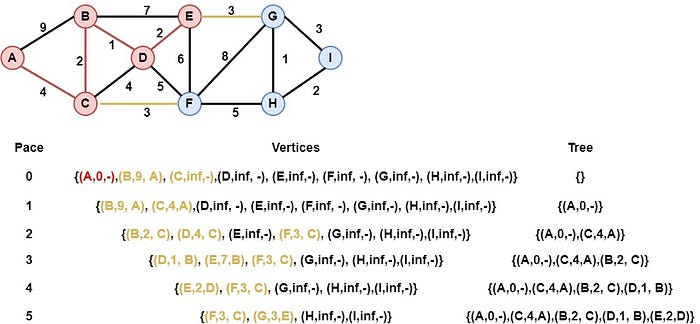Step 7: Vertex F is inserted into the tree

Vertex F is inserted into the tree, as it has the lowest value of distance, and through vertex F we are able to reach the vertex H with a cost of 5.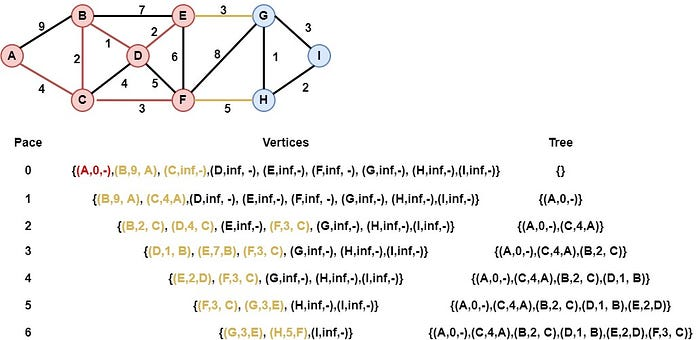Step 8: Vertex G is inserted into the tree

Vertex G is inserted into the tree, and through G we are now able to reach vertex H with cost 1 and vertex I with cost 3.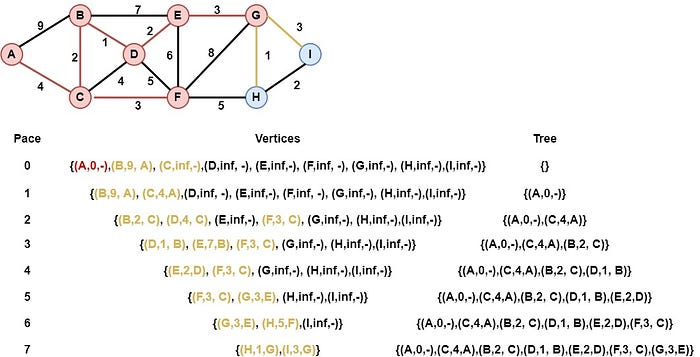Step 9: Vertex H is inserted into the tree

Vertex H is inserted into the tree, and through H we have found a better way to reach vertex I with cost 2.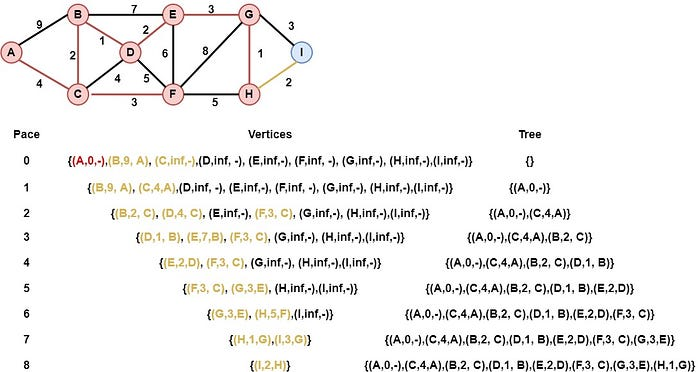Step 10: Vertex I is inserted into the tree

Finally, vertex I is inserted into the tree, and the algorithm terminates as the vertices structure is empty. The returned result is the following: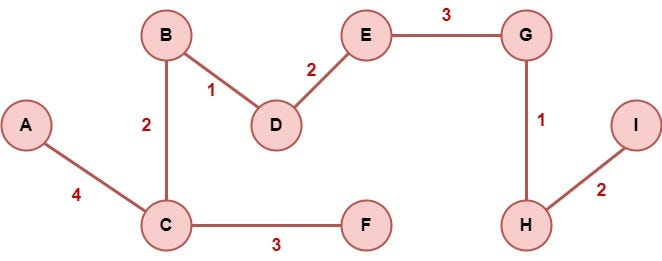### Python Implementation

Having understood the algorithmic procedure behind Prim's algorithm, we are ready to implement the algorithm in Python. We are going to extend the code from the Graphs article.

Firstly, we will update the class Node that represents a vertex in the graph and contains attributes about the value of the node, the neighbors, the length from the previous node, the previous node, and an indicator of whether the node has been visited or not. Also, the class is equipped with appropriate methods, such as the add_neighbor() method to add a new neighbor to the node, etc. An overview of the class Node is the following: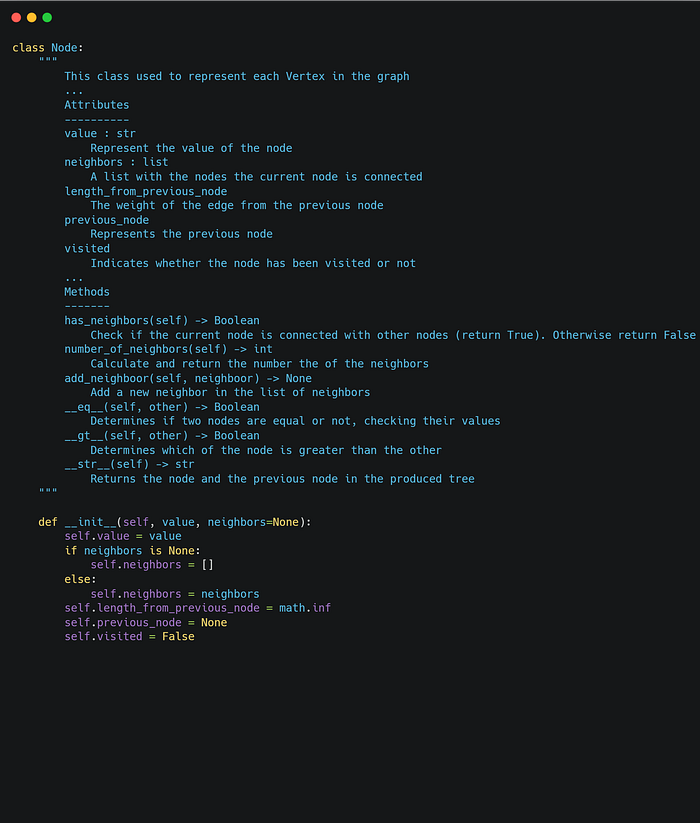Next, we define the class Graph, which represents the given graph of the problem. This class consists of a number of nodes, while it is equipped with necessary methods, such as the add_edge() method which insert a new edge between two given nodes in the graph. An overview of the class is the following: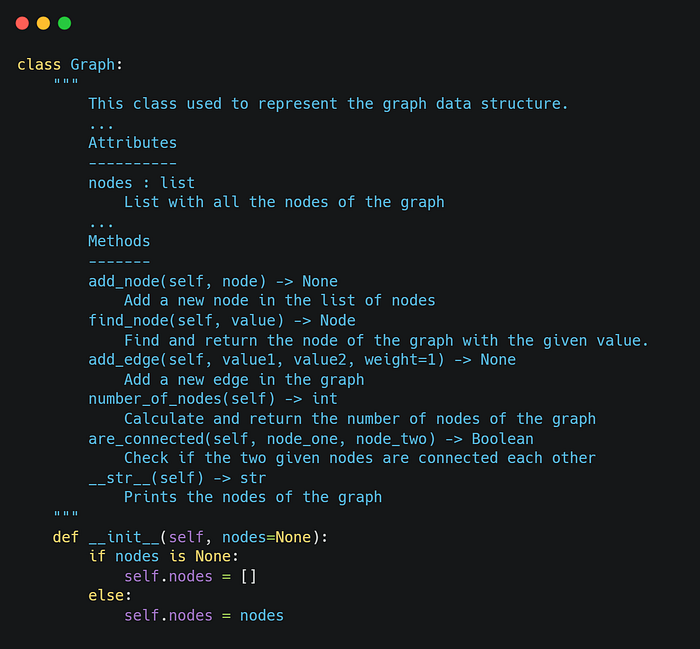Finally, we define the class Prim that represents Prim's algorithm. This class has various attributes such as the graph, the start node, the tree, and the vertices list. Also, it is equipped with methods such as the calculate_total_cost() method, which calculates the total cost of the produced tree, and the execution() method which is the algorithmic procedure of Prim's algorithm. Disclaimer: Even if Prim's algorithm starts from a random vertex, in our implementation we define the starting node. An overview of the class is the following: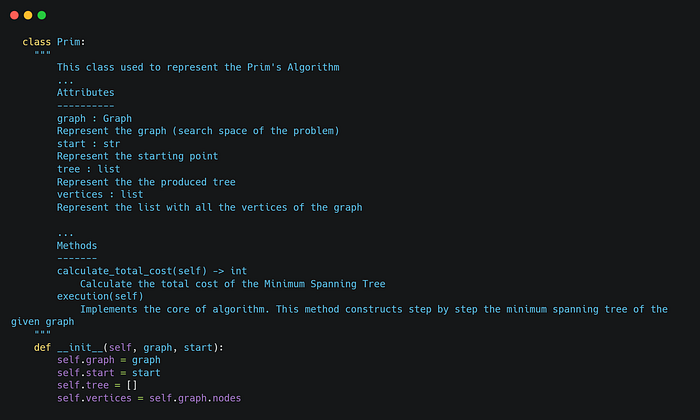The core algorithm of Prim is included in the execution method, that we can see below: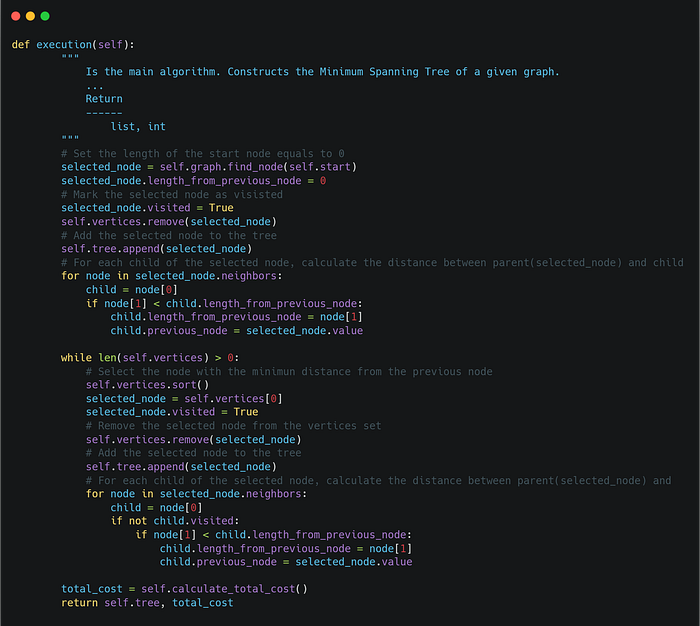### Example in Python

Having understood and implemented the algorithm in Python, we are ready to use it in order to find the Minimum Spanning Tree of a given graph. We are going to check if we have found the correct tree in the pen and paper example. Thus, we are going to model and execute the previous example as follows: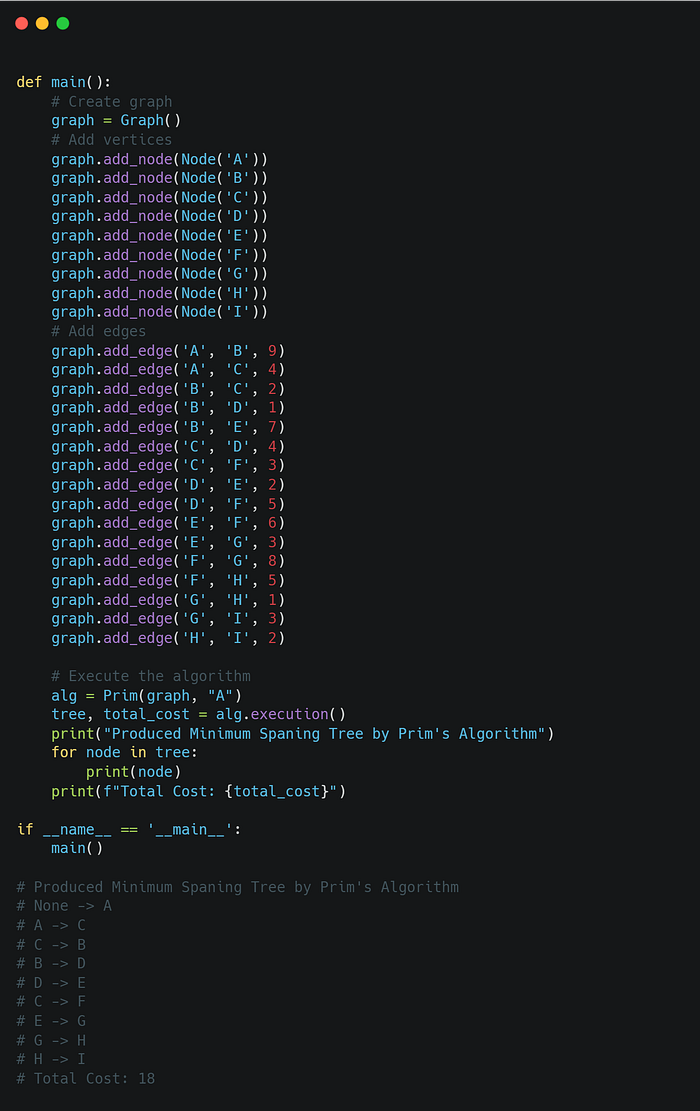As we can see, the algorithm returns the same results. Try to practice solving various examples using pen and paper and then verify your results using the above code.

### Conclusion

In this article, we had the opportunity to talk about Prim's algorithm, to find the Minimum Spanning Tree in a given graph. So, another useful algorithm is added to our toolbox. In the future, we will have the opportunity to talk about other graph algorithms, such as the Bellman-Ford algorithm. Until then, keep learning and keep coding. Thanks for reading.